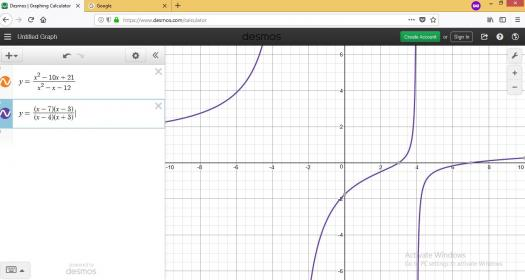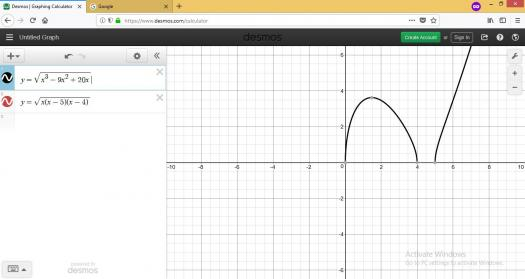# math

2 posts / 0 new
John Rey Arella...math

Guys can you help me to solve and graph this one . thanks

1. y^2=(x^2=1)(x-3)/X^3
2. x^2y-xy-x^2+10x-12y-21=0
3. y^2=x^3-9x^2+20x

fitzmerl duronI believe, you mean that we need to solve for $y$ and get their graphs...

For #1:

$$y^2 = \frac{(x^2-1)(x-3)}{x^3}$$ $$y^2 = \frac{x^3 -3x^2 -x +3}{x^3}$$ $$y^2 = 1 - \frac{3}{x} - \frac{1}{x^2} + \frac{3}{x^3}$$ $$y = \sqrt{1 - \frac{3}{x} - \frac{1}{x^2} + \frac{3}{x^3}}$$

The graphs of $\color{red}{y = \sqrt{\frac{(x^2-1)(x-3)}{x^3}}}$ and $\color{green}{y = \sqrt{1 - \frac{3}{x} - \frac{1}{x^2} + \frac{3}{x^3}}}$ is:Note that the green graph was superimposed by the red graph...that's why you only see a red graph...

For #2:

$$x^2y - xy - x^2 + 10x - 12y - 21 = 0$$ $$x^2y - xy - 12y = x^2 - 10x + 21$$ $$y(x^2 - x -12) = x^2 - 10x + 21$$ $$y = \frac{x^2 - 10x + 21}{x^2 - x -12}$$ $$y = \frac{(x-7)(x-3)}{(x-4)(x+3)}$$

The graphs of $\color{orange}{y = \frac{x^2 - 10x + 21}{x^2 - x -12}}$ and $\color{violet}{y = \frac{(x-7)(x-3)}{(x-4)(x+3)}}$ is:Note that the orange graph was superimposed by the violet graph...that's why you only see a violet graph...

For #3:

$$y^2 = x^3 - 9x^2 + 20x$$ $$y^2 = x(x^2 - 9x + 20)$$ $$y^2 = x(x-5)(x-4)$$ $$y = \sqrt{x(x-5)(x-4)}$$

The graphs of $\color{black}{y = \sqrt{x^3 - 9x^2 + 20x}}$ and $\color{pink}{y = \sqrt{x(x-5)(x-4)}}$ is:Note that the pink graph was superimposed by the black graph...that's why you only see a black graph...

Alternate solutions are encouraged.....:-)

• Mathematics inside the configured delimiters is rendered by MathJax. The default math delimiters are $$...$$ and $...$ for displayed mathematics, and $...$ and $...$ for in-line mathematics.